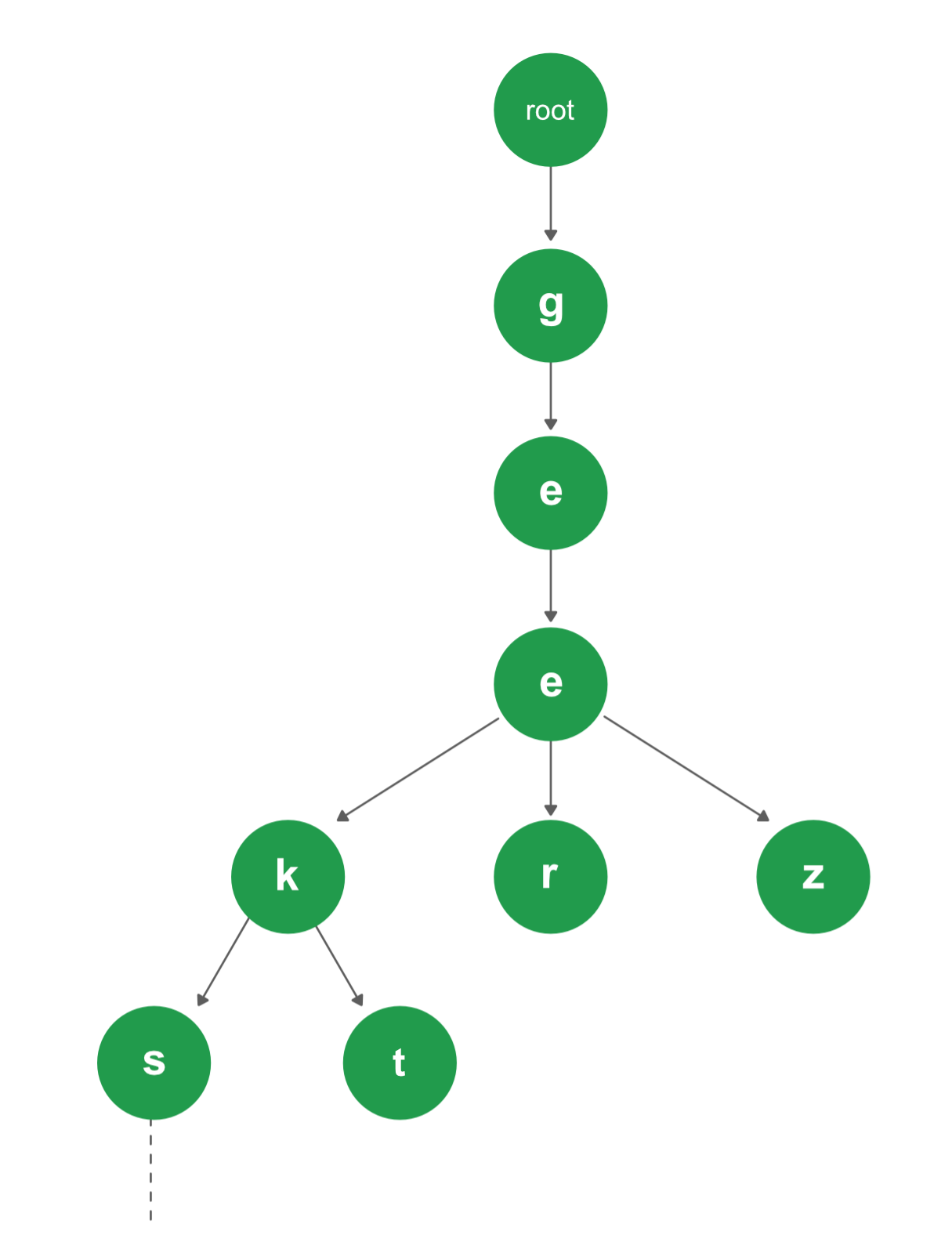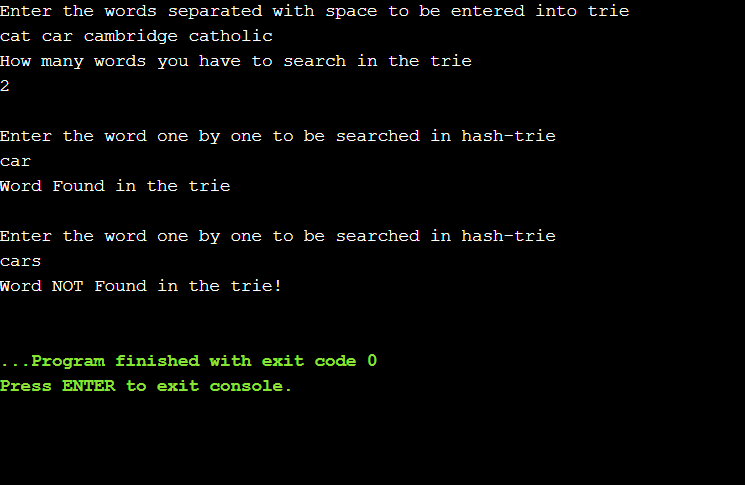# Java Program to Implement Hash Trie

• Last Updated : 02 Feb, 2021

A trie isn’t something CS students might have spent that much time on in college but it’s really very important for interviews. A trie is a data structure that is actually a type of tree, but it’s often used to store an associative array or a dynamic set where the keys are usually characters or strings, and its position in the tree defines the key with which it is associated. The way that it works is that every successor of a node have a common prefix of the string associated with that node which means each node might store a, just a character as its data but then if we look at the path from the root down to that node, that note is really representing a word or a part of a word, and so what allows us to do is, very quick lookups of a particular kind of word or character.Attention reader! Don’t stop learning now. Get hold of all the important Java Foundation and Collections concepts with the Fundamentals of Java and Java Collections Course at a student-friendly price and become industry ready. To complete your preparation from learning a language to DS Algo and many more,  please refer Complete Interview Preparation Course.

Java Code:

• Importing required module:

## Java

 `import` `java.io.*;``import` `java.util.*;`
• Now, we will make a class TrieHash in which we will implement a HashMap, it will also contain two constructors with zero and single array argument, a function to add characters to hash trie and a function to search for the specific string in the hash trie.

## Java

 `class` `TrieHash {`` ` `    ``// implementing a HashMap``    ``private` `HashMap origin;`` ` `    ``// implementing a zero-argument constructor``    ``public` `TrieHash()``    ``{``        ``// creating a new HashMap``        ``origin = ``new` `HashMap();``    ``}`` ` `    ``// implementing another constructor``    ``// with an array as a parameter``    ``public` `TrieHash(String[] array)``    ``{``        ``origin = ``new` `HashMap();``        ``// attaching that array string in the trie``        ``for` `(String c : array)``            ``attach(c);``    ``}`` ` `    ``// attach function to add character to the trie``    ``public` `void` `attach(String str)``    ``{``        ``HashMap node = origin;``        ``int` `i = ``0``;``        ``while` `(i < str.length()) {``            ``// if node already contains that key,``            ``//  we will simply point that node``            ``if` `(node.containsKey(str.charAt(i))) {``                ``node = node.get(str.charAt(i));``            ``}``            ``else` `{`` ` `                ``// else we will make a new hashmap with``                ``// that character and then point it.``                ``node.put(str.charAt(i),``                         ``new` `HashMap());``                ``node = node.get(str.charAt(i));``            ``}``            ``i++;``        ``}`` ` `        ``// putting 0 to end the string``        ``node.put(``'\0'``, ``new` `HashMap(``0``));``    ``}`` ` `    ``// function to search for the specific``    ``// string in the hash trie``    ``public` `boolean` `search(String str)``    ``{``        ``HashMap presentNode = origin;``        ``int` `i = ``0``;``        ``while` `(i < str.length()) {`` ` `            ``// will search for that character if it exists``            ``if` `(presentNode.containsKey(str.charAt(i))) {``                ``presentNode``                    ``= presentNode.get(str.charAt(i));``            ``}``            ``else` `{``                ``// if the character does not exist``                ``// that simply means the whole string``                ``// will also not exists, so we will``                ``// return false if we find a character``                ``// which is not found in the hash trie``                ``return` `false``;``            ``}``            ``i++;``        ``}``        ``// this will check for the end string,``        ``// and if the whole string is found,``        ``// it will return true else false``        ``if` `(presentNode.containsKey(``'\0'``)) {``            ``return` `true``;``        ``}``        ``else` `{``            ``return` `false``;``        ``}``    ``}``}`

Now all the required functions are coded, now we will test those functions with the user input. For this, we will again make a new class to test our hash trie which will prompt the user to enter the words for trie and the word to be searched for.

## Java

 `public` `class` `Main {``    ``// unreported exception IOException must be caught or``    ``// declared to be thrown``    ``public` `static` `void` `main(String[] args)``        ``throws` `IOException``    ``{``        ``BufferedReader br``            ``= ``new` `BufferedReader(``new` `InputStreamReader(``                ``System.in)); ``// this will accepts the words``                             ``// for the hash trie``        ``System.out.println(``            ``"Enter the words separated with space to be entered into trie"``);`` ` `        ``// will read the line which user will``        ``// provide with space separated words``        ``String input = br.readLine();`` ` `        ``// it will split all the words``        ``// and store them in the string array``        ``String[] c = input.split(``" "``);`` ` `        ``// now we will use constructor with string array as``        ``// parameter and we will pass the user entered``        ``// string in that constructor to construct the hash``        ``// trie``        ``TrieHash trie = ``new` `TrieHash(c);``        ``System.out.println(``            ``"\nEnter the word one by one to be searched in hash-trie"``);``        ``String word = br.readLine();`` ` `        ``// this will search for the word in out trie``        ``if` `(trie.search(word)) {``            ``System.out.println(``"Word Found in the trie"``);``        ``}``        ``else` `{``            ``System.out.println(``                ``"Word NOT Found in the trie!"``);``        ``}``    ``}``}`

Below is the implementation of the problem statement:

## Java

 `import` `java.io.*;``import` `java.util.*;`` ` `class` `TrieHash {`` ` `    ``// implementing a HashMap``    ``private` `HashMap origin;`` ` `    ``// implementing a zero-argument constructor``    ``public` `TrieHash()``    ``{`` ` `        ``// creating a new HashMap``        ``origin = ``new` `HashMap();``    ``}`` ` `    ``// implementing another constructor``    ``// with an array as a parameter``    ``public` `TrieHash(String[] array)``    ``{``        ``origin = ``new` `HashMap();``        ``// attaching that array string in the trie``        ``for` `(String c : array)``            ``attach(c);``    ``}`` ` `    ``// attach function to add``    ``// character to the trie``    ``public` `void` `attach(String str)``    ``{``        ``HashMap node = origin;``        ``int` `i = ``0``;``        ``while` `(i < str.length()) {``            ``// if node already contains thatkey,``            ``// we will simply point that node``            ``if` `(node.containsKey(str.charAt(i))) {``                ``node = node.get(str.charAt(i));``            ``}``            ``else` `{``                ``// else we will make a new hashmap with``                ``// that character and then point it.``                ``node.put(str.charAt(i),``                         ``new` `HashMap());``                ``node = node.get(str.charAt(i));``            ``}``            ``i++;``        ``}`` ` `        ``// putting 0 to end the string``        ``node.put(``'\0'``, ``new` `HashMap(``0``));``    ``}`` ` `    ``// function to search for the``    ``// specific string in the hash trie``    ``public` `boolean` `search(String str)``    ``{``        ``HashMap presentNode = origin;``        ``int` `i = ``0``;``        ``while` `(i < str.length()) {``            ``// will search for that character``            ``// if it exists``            ``if` `(presentNode.containsKey(str.charAt(i))) {``                ``presentNode``                    ``= presentNode.get(str.charAt(i));``            ``}``            ``else` `{``                ``// if the character does notexist that``                ``// simply means the whole string will``                ``// also not exists, so we will return``                ``// false if we find a character which``                ``// is not found in the hash trie``                ``return` `false``;``            ``}``            ``i++;``        ``}``        ``// this will check for the end string,``        ``// and if the whole string is found,``        ``// it will return true else false``        ``if` `(presentNode.containsKey(``'\0'``)) {``            ``return` `true``;``        ``}``        ``else` `{``            ``return` `false``;``        ``}``    ``}``}`` ` `public` `class` `Main {``    ``// unreported exception IOException``    ``// must be caught or declared to be thrown``    ``public` `static` `void` `main(String[] args)``        ``throws` `IOException``    ``{`` ` `        ``// this will accepts the words for the hash trie``        ``BufferedReader br = ``new` `BufferedReader(``            ``new` `InputStreamReader(System.in));``        ``System.out.println(``            ``"Enter the words separated with space to be entered into trie"``);``        ``// will read the line which user will``        ``// provide with space separated words``        ``String input = br.readLine();`` ` `        ``// it will split all the words and``        ``// store them in the string array``        ``String[] c = input.split(``" "``);`` ` `        ``// now we will use constructor with string``        ``// array as parameter and we will pass the``        ``// user entered string in that constructor``        ``// to construct the hash trie``        ``TrieHash trie = ``new` `TrieHash(c);``        ``System.out.println(``            ``"How many words you have to search in the trie"``);``        ``String count = br.readLine();``        ``int` `counts = Integer.parseInt(count);``        ``for` `(``int` `i = ``0``; i < counts; i++) {``            ``System.out.println(``                ``"\nEnter the word one by one to be searched in hash-trie "``);``            ``String word = br.readLine();``            ``// this will search for the word in out trie``            ``if` `(trie.search(word)) {``                ``System.out.println(``                    ``"Word Found in the trie"``);``            ``}``            ``else` `{``                ``System.out.println(``                    ``"Word NOT Found in the trie!"``);``            ``}``        ``}``    ``}``}`

Output1:Output2:My Personal Notes arrow_drop_up